# Ratio, Proportion and Variation for CAT Exam 2020

The concept of ratio, proportion and variation involves change. To compare two quantities we use ratios. Statements of equality involving ratios are called proportions. And variations are problems written as proportions.

• The comparison of two numbers or quantities by division is known as the ratio. It is denoted by the symbol ‘:’
• The correspondence of two ratios is called proportion. It is denoted by the symbol ‘::’
• Variations deal with how one quantity changes with respect to one or more other quantities.

Ratio and proportion and variation are one of the easiest topics in CAT Quantitative Aptitude section. Questions from this chapter are asked in conjunction with other concepts like mixtures and alligations, similar triangles, etc. Therefore the fundamental concepts of ratio and proportion and variation are not just important from a stand-alone perspective, but also to solve questions from other chapters.

## Ratio

The concepts of ratio are used in our day to day life. A ratio is a fraction which represents the relationship between two similar quantities (ones with the same units). Two ratios are said to be equal if their fractions are equivalent fractions when written in fractional form. Ratios can be compared and solved using cross multiplication. The ratios are equal if the cross products are equal.

A ratio depicts how many times one number is of another. If we have two terms p and q, with values 4 and 5 respectively, the ratio is depicted as p:q, i.e. 4:5 and is measured as p/q = 4/5. ‘p’ is called the antecedent and ‘q’ is called the consequent. There are two types of ratios:

Proper Ratio: When the antecedent is less than the consequent (numerator < denominator)

Example: 3:7, 1:2

Improper Ratio: When the antecedent is more than the consequent (numerator > denominator)

Example: 7:3, 5:2

Question: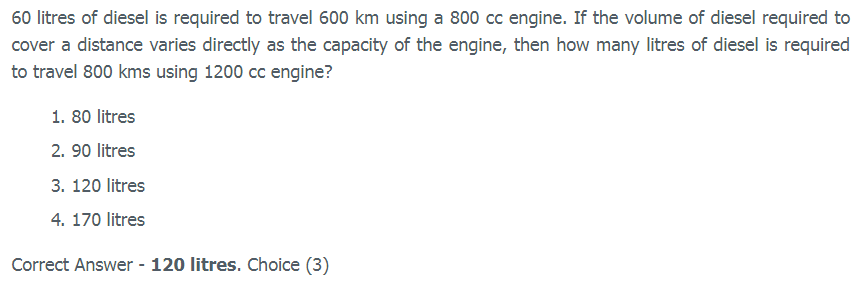Solution: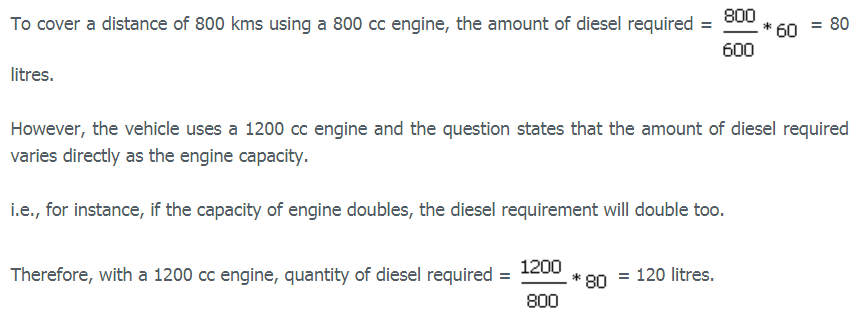## Proportion

A proportion is an arithmetic statement equating two ratios. Ratio and proportions are said to be faces of the same coin. Two ratios are said to be in proportion when they are equal in value. Visit properties of ratio and proportion to learn various important properties. Proportion is denoted by the symbol ‘=’ or ‘::’.

For example, Jack has 4 pets- a fish, a bird, a cat, and a dog. Now, what proportion of pets are 4 legged. Only 2 pets (the cat and the dog) have 4 legs. So the proportion of pets with 4 legs is 2/4 or ½.

In proportion two ratios, say p:q and x:y are called as means and extremes.

Example –  x:y :: p:q

Here the ratio x:y is termed as means and p:q as extremes.

Question: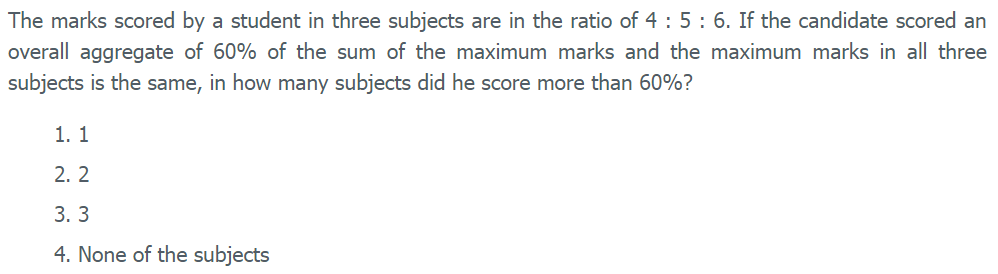Solution: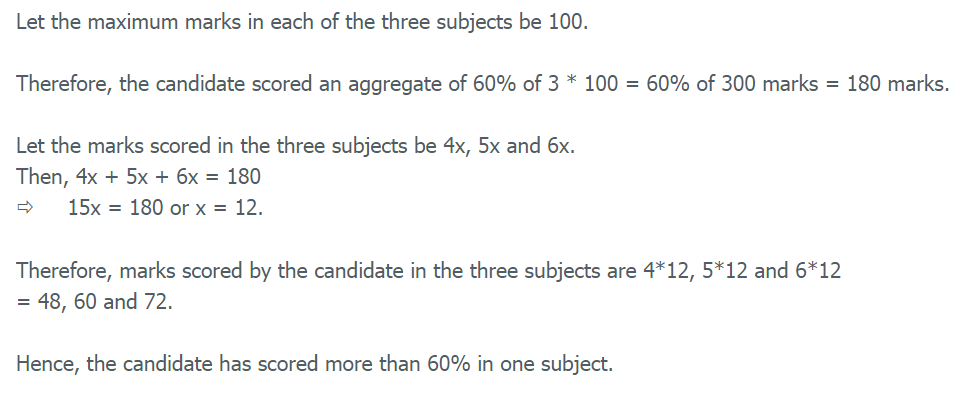## Variations

Variation deals with how one quantity changes with respect to one or more other quantities. Two quantities are said to vary with each other, if there exists a relationship between them such that a change is ‘A’ entails a change in ‘B’ and vice-versa.

There are two types of variation: direct variation and inverse variation.

Direct Variation

If Quantity 1 varies directly with Quantity 2; i.e. Quantity 2 increases if Quantity 1 increases and Quantity 2 decreases if Quantity 1 decreases;

then they are said to be in direct variation. For example, if Time is constant, the Speed will be directly proportional to the distance. That is, if the Speed is more, then the distance covered will also be more, and vice-versa Speed α Distance

Inverse variation

If Quantity 1 varies inversely with Quantity 2, then they are said to be in inverse variation. For example, Speed varies inversely with time; that is if the Speed is more, then the time taken to cover a constant distance will be less. This means that when speed is minimum, then time is maximum; and when speed is maximum, then time is minimum.

Question: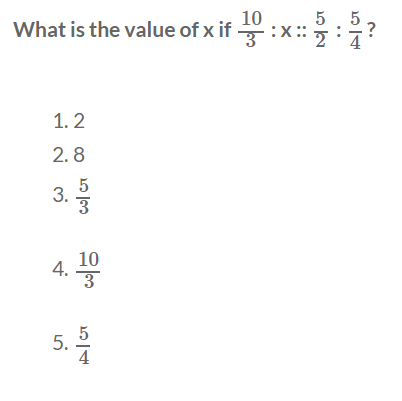Solution: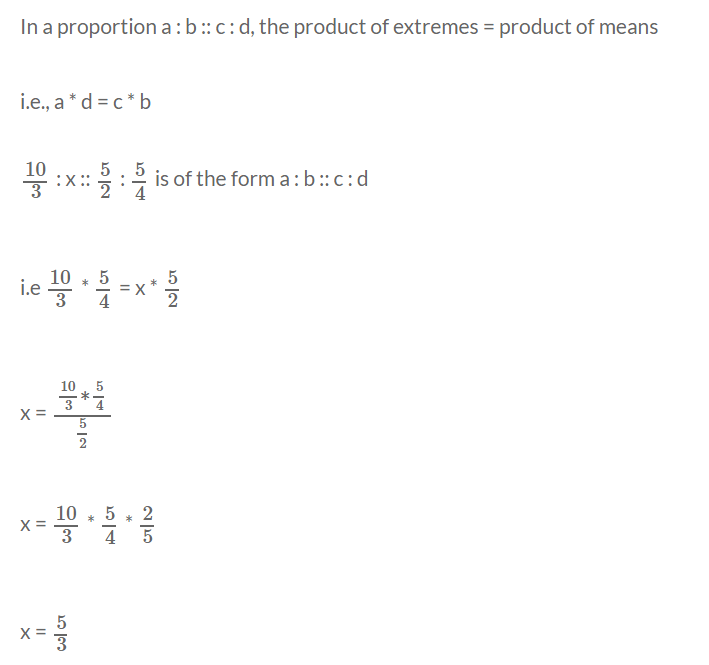Stay tuned with Byju’s to get the latest notification on CAT. solve CAT sample papers and previous year’s questions at Byju’s.